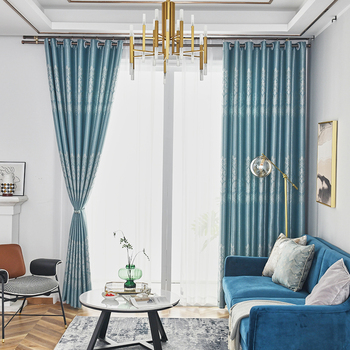# 智能窗帘控制系统(基于51单片机的自动窗帘控制系统)

• A+

1.简介

2.硬件设计

（1）光照检测电路

（2）灯光驱动电路

（3）步进电机驱动电路

（4）按键电路

（5）DS1302时钟电路

（5）总体电路图

3.软件设计

（1）主程序设计

void main(){ init_eepom(); //初始化EEPROM init_1602(); //lcd1602初始化 init_1602_dis_csf(); //lcd1602初始化显示 init_ds1302_io(); //初始化ds1302Io init_time0(); //初始化定时器 menu_1_break(); //菜单初始界面 init_ds1302(); //ds1302初始化 temperature1 = read1_temp(); //读温度1 delay_1ms(650); while(1) { key(); //按键程序 if(key_can < 10) { key_with(); } shoudong_kaiguan(); //手动开关窗帘 if(flag_200ms == 1) { flag_200ms = 0; temperature1 = read1_temp(); //读温度1 环境温度 if(temperature1 >= 99) temperature1 = 99; zinengchuanglian_dis(); //智能窗帘处理函数 guanxian = ad0832read(1,0) * 9.0 / 255; if((menu_1 == 0)) { read_time(); //读时间 init_1602_ds1302(); //显示时钟 write_sfm_18b20(2,13,temperature1); //显示温度 write_sfm1(2,0,guanxian); //显示温度 if(guanxian <= guanxian_set) LED=0; else LED=1; if(temperature1 >= t_high) beep = ~beep; //温度报警 ，蜂鸣器报警 else beep = 1; } } bujindj(); //步进电机函数 }}（2）步进电机驱动程序设计

void bujindj() //步进电机函数{ static uchar i; if(flag_z_f != 0) { if(flag_z_f == 1) //开 { if(bjdj_value >= 254) { flag_z_f = 0; bjdj_value = 254; P2 = P2 & 0xf0; //让4个IO口都不输出 write_eepom(); //保存 }else bjdj_value ++; } if(flag_z_f == 2) //关 { if(bjdj_value <= 1) { flag_z_f = 0; bjdj_value = 1; P2 = P2 & 0xf0; //让4个IO口都不输出 write_eepom(); //保存 }else bjdj_value --; } for(i=0;i<4;i++) //4相 { if(flag_z_f == 1) //开 P2=zheng[i];// & (P2 | 0xf0); // else if(flag_z_f == 2)//关 P2=fan[i];// & (P2 | 0xf0); // delay_uint(500); //改变这个参数可以调整电机转速 } }}由于代码较多，其他子程序就不一一介绍，有兴趣可下载源码去看看。

### 智能窗帘控制系统相关文章